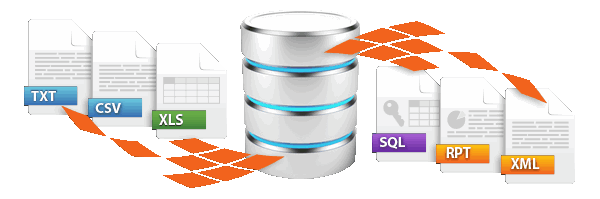Dynamic data import / exportsimply more productive
Up to 100,000 records can be processed with the freeware version!

# MS Excel CSV import using VB.NET

This describes a brief Visual Basic .NET example to illustrate how the .NET DataTable Adapter can be used to integrate any data source and target in FlowHeater. Of course the example is also provided as Visual Studio Solution source code.

## .NET DataTable Excel CSV import

Note: This example only serves as a demonstration. In fact this Excel import/export would be much simpler using with the Excel Adapter alone. If you want to repeat this example you will need Microsoft Excel 97 (or above) installed on your computer.

Once you have opened this example’s Definition, the screen should look something like the screenshot above. The READ side contains nothing of special note, it simply inputs a small CSV text file called "MS-Excel-CSV-Import.txt". Let us inspect the WRITE side’s Configurator. The Excel-Import.dll contains only one Visual Basic .NET function, which stores the data received on the WRITE side at the end of an Excel workbook called "Output.xls". If this workbook does not already exist, it is first created.

Try running this Definition and also inspect the VB .NET source code. You will find the Visual Studio Solution in the directory "Examples\EN\DataTableAdapter\VSExcelImport".

### VB.NET Code for the Excel import

Public Sub FlowHeater_SetDataTable(ByRef dt As DataTable)

If (dt.Rows.Count = 0) Then

' test call from the FlowHeater Designer or nothing to do

Exit Sub
End
If

Dim
excel As Object

'create Excel object
excel = CreateObject(
"Excel.Application")

'excel.Visible = True

Dim filename As String
filename = Path.Combine(System.Environment.CurrentDirectory,
"Output.xls")

' if not exist create

If File.Exists(filename) Then
excel.Workbooks.Open(filename)

Else
excel.ActiveWorkbook.SaveAs(filename)

End If

Dim
workbook As Object
workbook = excel.ActiveWorkbook

If workbook.Worksheets.Count() = 0 Then

End If

Dim
worksheet As Object
worksheet = workbook.Worksheets(1)

Dim nRow As Integer
nRow = 1

' search for first free row

Do While True
Dim
range As Object
range = worksheet.Cells(nRow, 1)

Dim cellValue As String
cellValue =
String.Empty

If range.Value2 <> Nothing Then

cellValue = range.Value2.ToString()

End If

If
cellValue.Length() = 0 Then
Exit
Do
End
If

nRow = nRow + 1

Loop

Dim
dr As DataRow

For Each dr In dt.Rows

Dim col As DataColumn

Dim nCol As Integer
nCol = 1

For Each col In dt.Columns
worksheet.Cells(nRow, nCol) = dr(col)
nCol = nCol + 1

Next col
nRow = nRow + 1

Next dr

workbook.Save()
excel.Quit()

End Sub

Microsoft®, Windows®, Excel® are registered trademarks of Microsoft Corporation